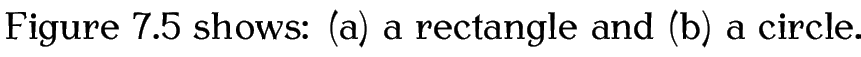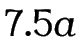# 7.4 Sub-Floats

Some floats have sub-floats within them. For example, a figure may contain several sub-figures, each of which requires a caption. The simplest way to do this is to use the subcaption package that provides the subfigure and subtable environments:

\begin{subfigure}[<pos>]{<width>}

\begin{subtable}[<pos>]{<width>}

Within these environments, you can use \caption to create a sub-caption. (In addition to the main \caption for the containing figure or table environment.)

Note:

The subcaption package requires the caption package, but doesn't automatically load it, so you'll need to load both:

\usepackage{caption,subcaption}

Example:

This is very similar to the side-by-side figures example from §7.1.1. Side-By-Side Figures.

\begin{figure}[htbp]
\begin{subfigure}[b]{0.5\linewidth}
\centering
\includegraphics{rectangle}
\caption{Rectangle}\label{fig:rectangle}
\end{subfigure}%
%
\begin{subfigure}[b]{0.5\linewidth}
\centering
\includegraphics{circle}
\caption{Circle}\label{fig:circle}
\end{subfigure}%
\caption{Two Shapes}
\label{fig:shape}
\end{figure}

This produces Figure 7.5. Elsewhere in the document, the figure and its components can be referenced:

Figure~\ref{fig:shapes2} shows some shapes. Figure~\ref{fig:rectangle} shows a rectangle and Figure~\ref{fig:circle} shows a circle.

which produces the following text:You can also reference just the sub-float using

\subref{<label>}

which is analogous to \ref, but only displays the sub-float number without the number associated with its containing float.

Example:

Figure~\ref{fig:shapes2} shows: (\subref{fig:rectangle}) a rectangle and (\subref{fig:circle}) a circle.

producesFigure 7.5: Two Shapes(a) Rectangle (b) Circle

Note:

The subfigure labels (a, b, etc) should typically be in italic . This can be achieved with the caption package using:

\DeclareCaptionLabelFormat{<format-name>}{<code>}

where <format-name> is the name for this new format and <code> is the code used to format the label where #2 gets replaced by the reference number.

Once you have defined a new format, you can then use

\captionsetup[<type>]{<options>}

to switch to that new format. For subfloats, <type> needs to be set to sub. The second argument <options> is a <key>=<value> comma-separated list. The key that sets the format is labelformat. (For further details about both \DeclareCaptionLabelFormat and \captionsetup, see the caption package documentation.)

For example, to create a format called em-noparens that displays the number in an emphasized font without parentheses:

Now switch to that new format:

\captionsetup[sub]{labelformat=em-noparens}

Note that this only changes the caption label format. It doesn't affect the font used by \ref or \subref. For \ref, you can use the fncylab package, which provides the command:

\labelformat{<ctr>}{<defn>}

Within <defn>, use #1 to represent the subfigure value and use \thefigure for the encapsulating figure number. For example:

Now

\ref{fig:circle}

will produceUnfortunately, this doesn't work for \subref. Instead you will have to do, for example, the following in the text:

\emph{\subref{fig:circle}}

If you want to add parentheses, the above can be modified to:

\DeclareCaptionLabelFormat{em-parens}{(\emph{#2})}
\captionsetup[sub]{labelformat=em-parens}
\labelformat{subfigure}{\thefigure(\emph{#1})}

For \subref, you will have to do, for example, the following in the text:

(\emph{\subref{fig:circle}})

Exercise 18: Creating Sub-Figures